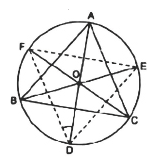# Bisectors of angles A, B and C of a triangle ABC intersect its circumcircle at D, E and F respectively. Prove that the angles of the triangle DEF are 90°-(1/2) A, 90°-(1/2) B and 90°-(1/2) C.Given:

∆ABC and it circum-circle AD,BE and CF are bisectors of ∠A,∠B and ∠C

Respectively.

To prove:∠ D=90°-1/2∠A , ∠E=90°-1/2∠B , ∠F=90°-1/2∠C

Construction: Join AE and AF.

Proof

∠FDA = ∠FCA ————-(i)

∠FDA = ∠EBA ————-(i)

By adding equations (i) and (ii) we get,

∠FDA+∠EDA = ∠FCA+∠EBA

Or, ∠FDE = ∠FCA+∠EBA = (½)∠C+(½)∠B

We know, ∠A +∠B+∠C = 180°

So, ∠FDE = (½)[∠C+∠B] = (½)[180°-∠A]

∠FDE = [90-(∠A/2)]

Similarly

∠FED = [90° -(∠B/2)] °

∠EFD = [90° -(∠C/2)] °